## RD Sharma Class 8 Solutions Chapter 14 Compound Interest Ex 14.4

These Solutions are part of RD Sharma Class 8 Solutions. Here we have given RD Sharma Class 8 Solutions Chapter 14 Compound Interest Ex 14.4

Other Exercises

Question 1.
The present population of a town is 28,000. If it increases at the rate of 5% per annum, what will be its population after 2 years ?
Solution:
Present population = 28000
Rate of increase (R) = 5% p.a.
Period (n) = 2 years
Population after 2 years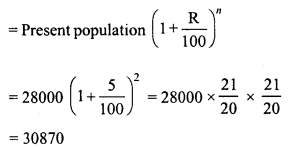Question 2.
The population of a city is 125000. If the annual birth rate and death rate are 5.5% and 3.5% respectively, calculate the population of city after 3 years.
Solution:
Present population = 125000
Rate of birth = 5.5%
and rate of death = 3.5%
Increase = 5.5 – 3.5 = 2% p.a.
Period = 3 years.
Population after 3 years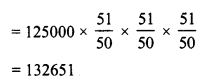Question 3.
The present population of a town is 25000. It grows at 4%, 5% and 8% during first year, second year and third year respectively. Find its population after 3 years.
Solution:
Present population = 25000
Increase in first year = 4%
in second year= 5% and
in third year = 8%
Population after 3 years =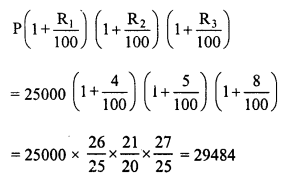Question 4.
Three years ago, the population of a town was 50000. If the annual increase during three successive years be at the rate of 4%, 5% and 3% respectively, find the present population.
Solution:
Three years ago,
Population of a town = 50000
Annual increase in population in first year = 4%
in second year = 5%
and in third year = 3%
Present population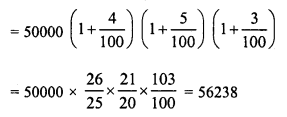Question 5.
There is a continuous growth in population of a village at the rate of 5% per annum. If its present population is 9261, what it was 3 years ago ?
Solution:
Let 3 years ago, population = P
Present population = 9261
Rate of increase (R) = 5% p.a.
Period (n) = 3 years.Question 6.
In a factory, the production of scooters rose to 46305 from 40000 in 3 years. Find the annual rate of growth of the production of scooters.
Solution:
Production of scooters 3 years ago (P) = 40000
Present production (A) = 46305
Period (n) = 3 years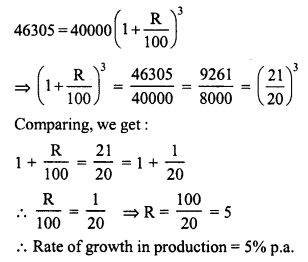Question 7.
The annual rate of growth in population of a certain city is 8%. If its present population is 196830, what it was 3 years ago ?
Solution:
Let 3 years ago, the population of a city = P
Rate of growth (R) = 8% p.a.
Present population = 196830
Period (n) = 3 years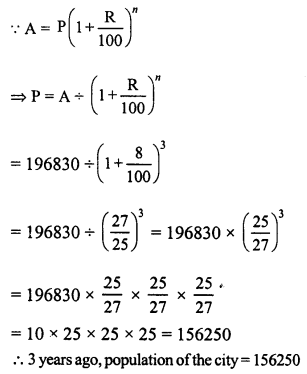Question 8.
The population of a town increases at the rate of 50 per thousand. Its population after 2 years will be 22050. Find its present population.
Solution:
Population after 2 years = 22050
Rate of increase = 50 per thousand
Period (n) = 2 years
Let present population = P, then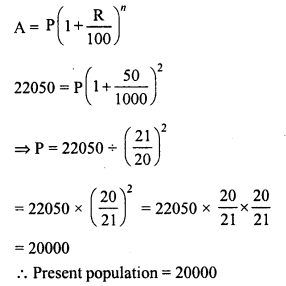Question 9.
The count of bacteria in a culture grows by 10% in the first hour, decreases by 8% in the second hour and again increases by 12% in the third hour. If the count of bacteria in the sample is 13125000, what will be the count of bacteria after 3 hours ?
Solution:
Present count of bacteria = 13125000
In first hour increase = 10%
decrease in second hour = 8%
increase in third hour = 12%
Count of bacteria after 3 hoursQuestion 10.
The population of a certain city was 72000 on the last day of the year 1998. During next year it increased by 7% but due to an epidemic it decreased by 10% in the following year. What was its population at the end of the year 2000 ?
Solution:
On the last day of 1998,
Population of a town = 72000
In the first year, increase = 7%
In the second year, decrease = 10%
Population in the last day of 2000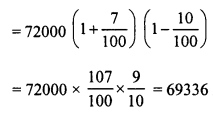Question 11.
6400 workers were employed to construct a river bridge in four years. At the end of the first year, 25% workers were retrenched. At the end of the second year, 25% of those working at that time were retrenched. However, to complete the project in time, the number of workers was increased by 25% at the end of the third year. How many workers were working during the fourth year ?
Solution:
Number of workers at the beginning = 6400
Period = 4 years.
At the end of 1st year, workers retrenched = 25%
At the end of second year, workers retrenched = 25%
At the end of third year, workers increased = 25%
Total number of workers during the 4 years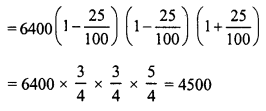Question 12.
Aman started a factory with an initial investment of Rs 1,00,000. In the first year, he incurred a loss of 5%. However, during the second year, he earned a profit of 10% which in the third year rose to 12%. Calculate his net profit for the entire period of three years.
Solution:
Initial investment = Rs 100000
Loss in the first year = 5%
Profit in the second year = 10%
Profit in the third year = 12%
Investment at the end of 3 yearsQuestion 13.
The population of a town increases at the rate of 40 per thousand annually. If the present population be 175760, what was the population three years ago ?
Solution:
Present population (A) = 175760
Increase rate = 40 per 1000
Period = 3 years
Let 3 years ago,
population was = PQuestion 14.
The production of a mixi company in 1996 was 8000 mixies. Due to increase in demand it increases its production by 15% in the next two years and after two years its demand decreases by 5%. What will be its production after 3 years ?
Solution:
Production of Mixi in 1996 = 8000
Increase in next 2 years = 15%
Decrease in the third year = 5%
Production after 3 years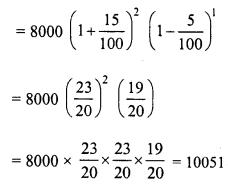Question 15.
The population of a city increases each year by 4% of what it had been at the beginning of each year. If the population in 1999 had been 6760000, find the population of the city in (i) 2001 (ii) 1997.
Solution:
Population of a city in 1999 = 6760000
Increase = 4%
(i) Population in 2001 is after 2 years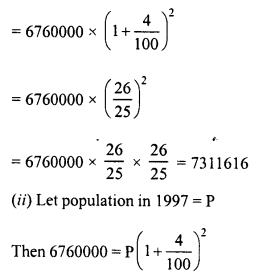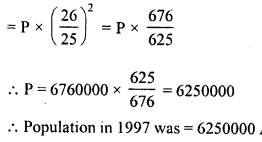Question 16.
Jitendra set up a factory by investing Rs 25,00,000. During the first two successive years his profits were 5% and f 10% respectively. If each year the profit was on previous year’s capital, compute his total profit.
Solution:
Investment in the beginning = Rs 25,00,000
Profit during the first 2 years = 5% and 10% respectively
Investment after 2 years will be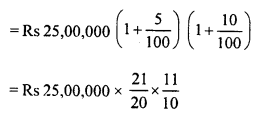= Rs 28,87,500
Amount of profit = Rs 28,87,500 – Rs 25,00,000 = Rs 3,87,500

Hope given RD Sharma Class 8 Solutions Chapter 14 Compound Interest Ex 14.4 are helpful to complete your math homework.

If you have any doubts, please comment below. Learn Insta try to provide online math tutoring for you.